In Machine Learning, a confusion matrix is a specific table layout that allows visualization of the performance of an algorithm. Each row of the matrix represents the instances in a predicted class while each column represents the instances in an actual class (or vice versa). The name stems from the fact that it makes it easy to see if the system is confusing two classes (i.e. commonly mislabeling one as another).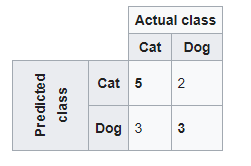Image 1: Example of a Confusion Matrix in Python Programming Language

On Image 1 we can see an example of a confusion matrix create for the problem of a classification system that has been trained to distinguish between cats and dogs.

The confusion matrix will summarize the results of testing the algorithm for further inspection. Assuming a sample of 13 animals of which 8 are cats and 5 are dogs.

In this confusion matrix, of the 8 actual cats, the system predicted that 3 were dogs, and of the 5 dogs, it predicted that 2 were cats.

All correct predictions are located in the diagonal of the table (highlighted in bold), so it is easy to visually inspect the table for prediction errors, as they will be represented by values outside the diagonal.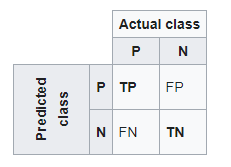Image 2: The concept of Confusion Matrix

On Image 2 we can see the concept of the confusion matrix. We can see different letters in the cells, here is what all of those letters mean:  P = positive; N = Negative; TP = True Positive; FP = False Positive; TN = True Negative; FN = False Negative.

Using these parameters, you can calculate different characteristics of the data you are working with like:

### – Accuracy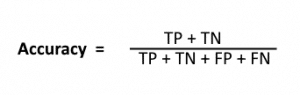There are problems with the accuracy since it takes in count that the costs are the same as the both types of errors.

### – Precision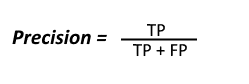A trivial way to have perfect precision is to make one single positive prediction and ensure it is correct (precision = 1/1 = 100%). This would not be very useful since the classifier would ignore all but one positive instance.

So precision is typically used along with another metric named recall, also called sensitivity or true positive rate (TPR): this is the ratio of positive instances that are correctly detected by the classifier.

High Precision indicates an example labeled as positive is indeed positive (a small number of FP).

### – Recall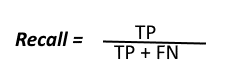Recall can be defined as the ratio of the total number of correctly classified positive examples divide to the total number of positive examples. High Recall indicates the class is correctly recognized (a small number of FN).

### – F1 Score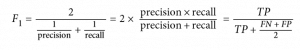This measure comes handy because it represents both Precision and Recall equally. The F1 score is the harmonic mean of precision and recall.

The regular mean treats all values equally and the harmonic mean gives much more weight to low values. As a result, the classifier will only get a high F1 score if both recall and precision are high.

We need them to be equally represented because of the following:

1. High recall, low precision which means that most of the positive examples are correctly recognized (low FN) but there are a lot of false positives.
2. Low recall, high precision which means that we miss a lot of positive examples (high FN) but those we predict as positive are indeed positive (low FP)

## Implementing Confusion Matrix using Python programming language

So, in this article, we are going to show you how to implement the confusion matrix and determine its measurements using the Python programming language, following the formulas in the previous section and using the Scikit-library.

We are going to use the example from the previous section where we had 13 animals, out of which 8 are cats and 5 are dogs.

from sklearn.metrics import confusion_matrix, accuracy_score, precision_score, recall_score, f1_score

#0 = cat, 1 = dog
actual = [0, 0, 1, 0, 1, 1, 0, 1, 0, 1, 0, 0, 0]
predicted = [0, 1, 1, 0, 0, 1, 0, 1, 0, 0, 0, 1, 1]
confusion_matrix = confusion_matrix(predicted, actual)

print (‘Confusion Matrix :’)
print(confusion_matrix)

TP = confusion_matrix
FP = confusion_matrix
FN = confusion_matrix
TN = confusion_matrix

print(“TP: “,TP, “FP: “, FP, “TN: “, TN, “FN: “,FN)

With the code above we import the metrics that we are going to need from the Scikit-learn library, and we prepare our data. As you can see, we have a list of actual values (0=cat and 1=dog) and we have a list of predicted values (0=cat and 1=dog).

If we have the same value for the same position in both arrays, that means that the system made the right prediction otherwise it made the wrong prediction.

Since we made these values by hand we made it look exactly like the example given in the previous section.

Here is the output of the code above:

Confusion Matrix :

[[5 2]

[3 3]]

TP:  5 FP:  2 TN:  3 FN:  3

As you can see, we have the same values, for the parameters as in the example.

accuracy_score_ = (TP+TN)/(TP+TN+FP+FN)
print(‘Accuracy Score using the formula: ‘, accuracy_score_)
print(‘Accuracy Score using Scikit-learn: ‘,accuracy_score(actual, predicted))

With this code, we are calculating the accuracy score. As you can see, you can do it, by following the formula or by using the Scikit-learn method. The results are these:

Accuracy Score using the formula: 0.6153846153846154
Accuracy Score using Scikit-learn: 0.6153846153846154

As you can see, the accuracy is identical, so you can use whatever you prefer.

precision_score_ = TP/(TP+FP)
print(‘Precision Score using the formula: ‘, precision_score_)
print(‘Precision score using Scikit-learn: ‘,precision_score(actual, predicted))

With the code above, we calculate the precision score. You can also do it by following the formula or using Scikit-learn methods. Here are the results:

Precision Score using the formula:  0.7142857142857143

Precision score using Scikit-learn:  0.5

We can see, that in this instance, the results are quite different. Now first we taught that is because we built the confusion matrix by adding first the predicted (rows) than the actual values (columns) with the code: confusion_matrix = confusion_matrix(predicted, actual), because we wanted to have the same look as in the example(default is to have actual as rows and predicted as columns), but that was not the reason, and we got the same results.

So when you are doing precision predictions, use the Scikit-learn, at the end of the day it is created by teams of experienced engineers.

But if you want to try it by following the formulas it is also cool, since you will make it custom solution.

recall_score_ = TP/(TP+FN)
print(‘Recall Score using the formula: ‘, recall_score_)
print(‘Recall Score using Scikit-learn: ‘, recall_score(actual, predicted))

Next is the recall score. As with the previous examples you can use the formula and create a custom solution, or you can use the Scikit-learn methods. Here are the results:

Recall Score using the formula: 0.625Recall

Score using Scikit-learn:  0.6

The results, in this case, are quite similar, but we still suggest you to use the Scikit-learn method, since again, it is created by experienced engineers and it is a long time on the market.

f1_score_ = TP/(TP+((FN+FP)/2))
print(‘F1 Score using the formula: ‘, f1_score_)

print(‘F1 Score using Scikit-learn: ‘,f1_score(actual,predicted))

The last one is the F1 score. We’ve used the formula and the Scikit-learn method to determine how close the results are going to be:

F1 Score using the formula:  0.6666666666666666

F1 Score using Scikit-learn:  0.5454545454545454

You can see that the difference is not that great (it’s 0.(12)), but again out suggestion is to go with Scikit-learn, that is what you are going to use in real projects.

## Conclusion

The confusion matrix is an easy way to determine if your algorithm makes a good prediction on the data you are using.

It takes no time to implement and it will give you fairy good results that will help you determine which way to go.

There are more complex techniques than this one, so in order to understand them, you need to start from the beginning, and the confusion matrix is a beginning as it gets.

As for the way of how you should implement it, we will say that if you are new to this, then first follow the formulas, and then use the library methods for better calculations.

If you are new, the formulas will help you remember how the results are produced, and what is dependent on what.

For this article, we were inspired by the book Hands-On Machine Learning with Scikit-Learn and TensorFlow: Concepts, Tools, and Techniques to Build Intelligent Systems by Aurelien Geron, some Wikipedia articles and articles from GeeksforGeeks.

If you are new to Machine Learning, Deep Learning or Data Science, and you want to learn them in a shorter time then here are some articles that you will find very useful and helpful:

Like with every post we do, we encourage you to continue learning, trying, and creating.